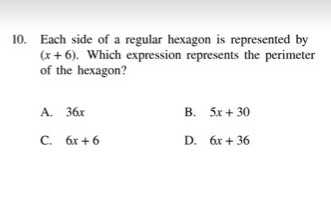### ¿Todavía tienes preguntas de matemáticas?

Pregunte a nuestros tutores expertos
Algebra
Pregunta10. Each side of a regular hexagon is represented by

$$( x + 6 )$$ . Which expression represents the perimeter of the hexagon?

A. $$36 x$$ B. $$5 x + 30$$

C. $$6 x + 6$$ D. $$6 x + 36$$